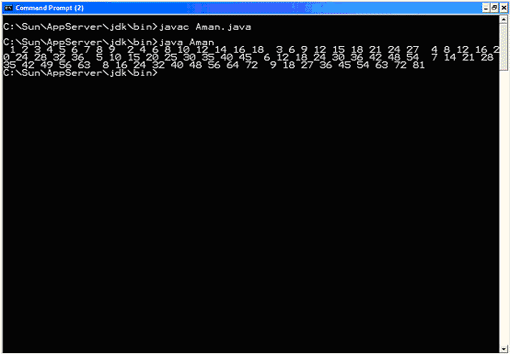## Core Java

Java Evolution
Overview of Java Language
Constant, Variable and Datatype
Operator and Expression
Decision making, Branching and Looping
Class Object and Method
Array and String
Inheritance
Package
 1) It Defines a block of code to be executed as long as a particular condition is met. The condition is tested prior to each iteration. It has the general syntax while (condition) { statements; } where condition represents a binary expression that, if true, permits an iteration of the loop. If no longer true, processing continues at the first statement after the closing brace of the while loop. The braces may be omitted if the loop consists of a single statement. This would constitute a "single statement while loop". 1) It does not provide for initialization of variables or automatic update expressions. In     spite of these limitations, a while loop can be used in place of nearly any for loop as     shown by these examples: Example 1: Counting to 10 without a local variable int i = 1; while (i <= 10) { System.out.println(i); i++; } Loop control variable i is initialized outside the loop. Prior to each iteration, the value of i is tested to determine if it is still less than or equal to 10. If so, the current value of i is displayed and i is incremented. Otherwise processing will jump to the first statement after the closing brace of the loop. Example 2: An endless loop while (true) System.out.println("I won't end"); This is the preferred technique for launching an endless loop. Example 3: A small program using nested while loops to generate a 9 x 9 multiplication table: import java.io.*; public class Aman { public static void main(String[] args) { // Initialize the row number. int row = 1; // This outer loop generates one row of the multiplication // table during each iteration. while (row <= 9) { // Initialize the column number. int column = 1; // This inner loop generates one column of the current row // of the multiplication table during each iteration. while (column <= 9) { // If a one digit number is about to be displayed, preceed it // with four spaces. Otherwise, preceed it with three spaces. if ((row * column) < 10) { System.out.print(" "); } else { System.out.print(" "); } // Display the number. System.out.print((row * column)); // Increment the column number. column++; } // End the current line. System.out.print(" "); // Increment the row number. row++; } } } Output: